## Chapter 4 triangles R.D. Sharma Solutions for Class 10th Math Exercise 4.5

Exercise 4.5

1. In fig. 4.136, ∆ACB ~ ∆APQ. If BC = 8 cm, PQ = 4 cm, BA = 6.5 cm and AP = 2.8 cm, find CA and AQ.Solution2. In Fig. 4.137, AB || QR. Find the length of PB.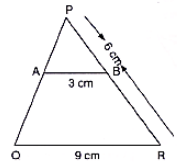Solution3. In fig. 4.138, XY || BC. Find the length of XY .Solution4. In a right angled triangle with sides a and b and hypotenuse c, the altitude drawn on the hypotenuse is x. Prove that ab = cx.

Solution5. In Fig. 4.139, ∠ABC = 90° and BD ⊥ AC. If BD = 8 cm and AD = 4 cm, find CD .Solution6. In Fig. 4.140, ∠ABC = 90° and BD ⊥ AC. If AB = 5.7 cm, BD = 3.8 cm and CD = 5.4 cm, find BC.Solution7. In Fig. 4.141, DE || BC such that AE = (1/4) AC. If AB = 6 cm, find AD .Solution8. In fig. 4.412, if AB ⟂ BC, DC ⟂ BC and DE ⟂ AC, prove that △CED ~ △ABC.Solution

Given,
AB ⟂ BC
DC ⟂ BC
So, Both AB and DC are perpendicular to same line BC.
We know that, two lines, perpendicular to same line are parallel.
∴ AB | | DC
Since, AC is transverse (from diagram) (i)
Now, In △ABC and △DCE
∠ABC = ∠DCE = 90° (Given AB⟂BC)
∠BAC = ∠DCE (from i)
Therefore, by AA criteria for similarity
△CED ~ △ABC .

9. Diagonals AC and BD of trapezium ABCD with AB || DC intersect each other at the point O. Using similarity criterion for two triangles, show that OA/OC = OB/OD .

Solution

It is given that trapezium ABCD with AB | | DC.O is the point of intersection of AC and BD .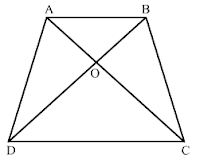We have to prove that OA/OC = OB/OD
Now, in △AOB and △COD
∠AOB = ∠COD (Vertically opposite angles)
∠OAB = ∠OCD (Alternate angles)
∴ △AOB ~ △COD (AA Similarity)
Hence, OA/OC = OB/OD (Corresponding sides are proportional)

10. If △ABC and △AMP are two right triangles, triangles, right angled at B and M respectively such that ∠MAP = ∠BAC. Prove that
(i) △ABC ~ △AMP
(ii) CA/PA = BC/MP

Solution(i) It is given that △ABC and △AMP are two right angle triangles,
Now, in △ABC and △AMP, we have
∠MAP = ∠BAC (Given)
∠AMP = ∠B = 90°
△ABC ~ △AMP (AA Similarity)

(ii) △ABC ~ △AMP
So, CA/PA = BC = MP (corresponding sides are proportional) .

11. A vertical stick 10 cm long casts a shadow 8 cm long. At the same time a shadow 30 m long. Determine the height of the tower.

Solution12. In below fig., ∠A = ∠CED, Prove that ∆CAB ~ ∆CED. Also, find the value of x.Solution13. The perimeters of two similar triangles are 25 cm and 15 cm respectively. If one side of first triangle is 9 cm, what is the corresponding side of the other triangle?

Solution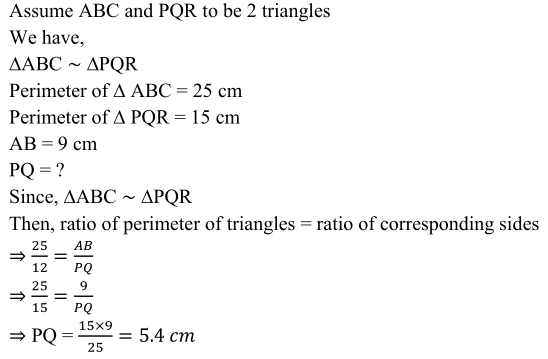14. In ∆ABC and ∆DEF, it is being given that: AB = 5 cm, BC = 4 cm and CA = 4.2 cm; DE =10cm, EF = 8 cm and FD = 8.4 cm. If AL ⊥ BC and DM ⊥ EF, find AL: DM.

Solution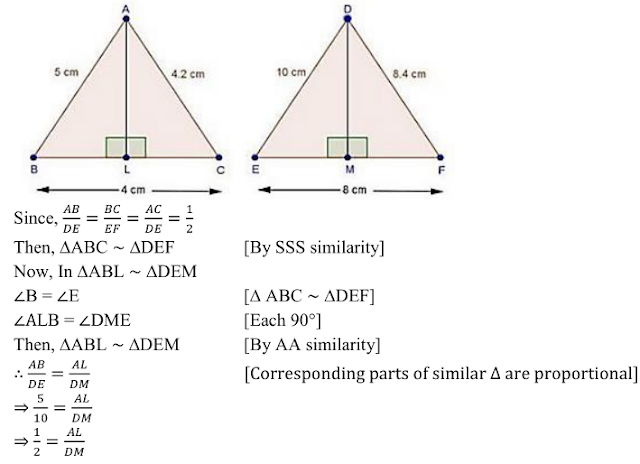15. D and E are the points on the sides AB and AC respectively of a ∆ABC such that: AD = 8 cm, DB = 12 cm, AE = 6 cm and CE = 9 cm. Prove that BC = 5/2 DE.

Solution16. D is the mid-point of side BC of a ∆ABC. AD is bisected at the point E and BE produced cuts AC at the point X. Prove that BE : EX = 3 : 1.

Solution17. ABCD is a parallelogram and APQ is a straight line meeting BC at P and DC produced at Q. Prove that the rectangle obtained by BP and DQ is equal to the AB and BC.

Solution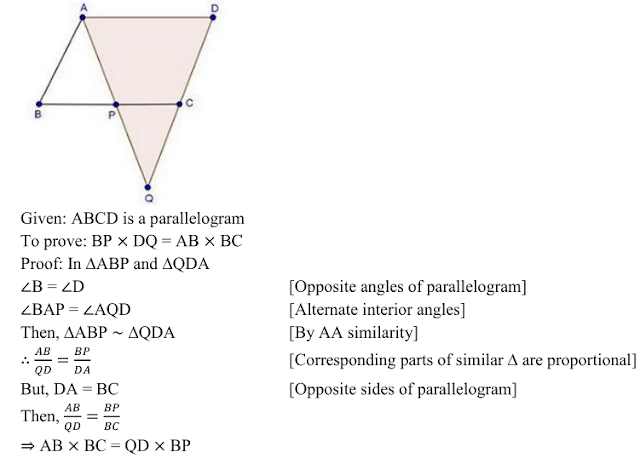18. In △ABC, AL and CM are the perpendiculars from the vertices A and C to BC and AB respectively . AL and CM intersect at O, prove that :
(i) △OMA and △OLC
(ii) OA/OC = OM/OL

Solution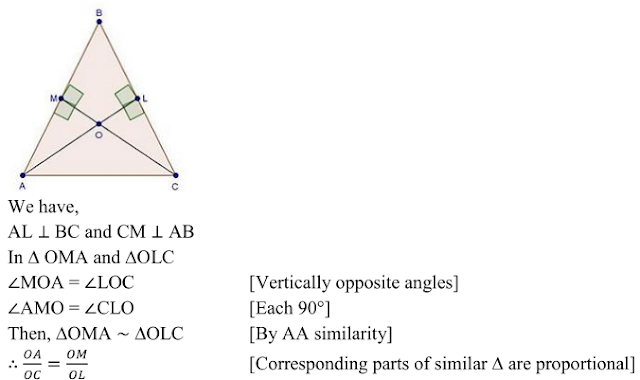19. ABCD is a quadrilateral in which AD = BC. If P, Q, R, S be the mid-points of AB, AC, CD and BD respectively, show that PQRS is a rhombus.

Solution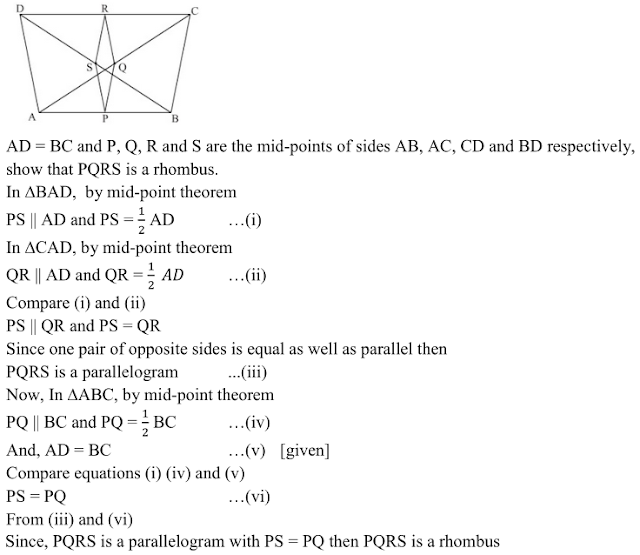20. In an isosceles ∆ABC, the base AB is produced both the ways to P and Q such that AP × BQ = AC2. Prove that △APC ~ △BCQ .

Solution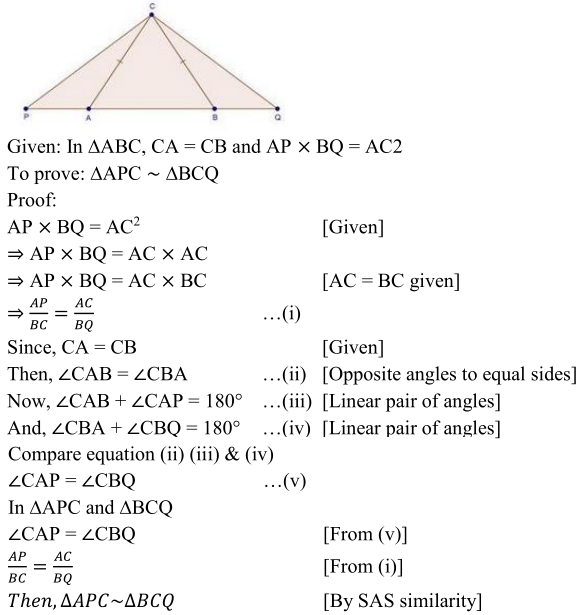21. A girl of height 90 cm is walking away from the base of a lamp-post at a speed of 1.2m/sec. If the lamp is 3.6 m above the ground, find the length of her shadow after 4 seconds.

Solution22. A vertical stick of length 6 m casts a shadow 4 m long on the ground and at the same time a tower casts a shadow 28 m long. Find the height of the tower.

Solution23. In below Fig., ∆ABC is right angled at C and DE ⊥ AB. Prove that ∆ABC ~ ∆ADE and Hence, find the lengths of AE and DE .Solution24. In fig., 4.142, PA, QB and RC are each perpendicular to AC. Prove that 1/x + 1/z + 1/y .Solution25. In Fig below we have AB || CD || EF. If AB = 6 cm, CD = x cm, EF = 10 cm, BD = 4 cm and DE = y cm, calculate the values of x and y.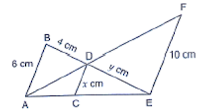Solution